# Overview

This tutorial will teach you and make you learn how to document sample Manual Test Cases for Calculator Windows Application.

In reality, we don’t write test cases for an application straight away. The actual process depends on many factors like SDLC methodology (System Development Life Cycle), Test Strategy, Organization, Input documents, etc.

## Input Documents

We need some documents to develop efficient and effective Test cases for an application under test(AUT).

Example Input Documents:

• Functional Requirements.
• SRS Document( Software Requirement Specification ).
• User stories, Actors, etc.

### Actors and User stories

https://www.testingdocs.com/windows-calculator-actors-and-user-stories/

## Windows Calculator

Windows Calculator is a standard default Windows Application (calc.exe ) under the folder

C:\Windows\System32

The Calculator application has many types:

• Standard
• Scientific
• Graphing
• Programmer
• Date Calculation

The standard calculator is limited to simple mathematical calculations. A scientific calculator is advanced with trigonometric, logarithmic functions, etc.  In this tutorial, let’s stick to the Standard Windows Calculator.

The Standard Windows Calculator UI on Windows 10 operating system: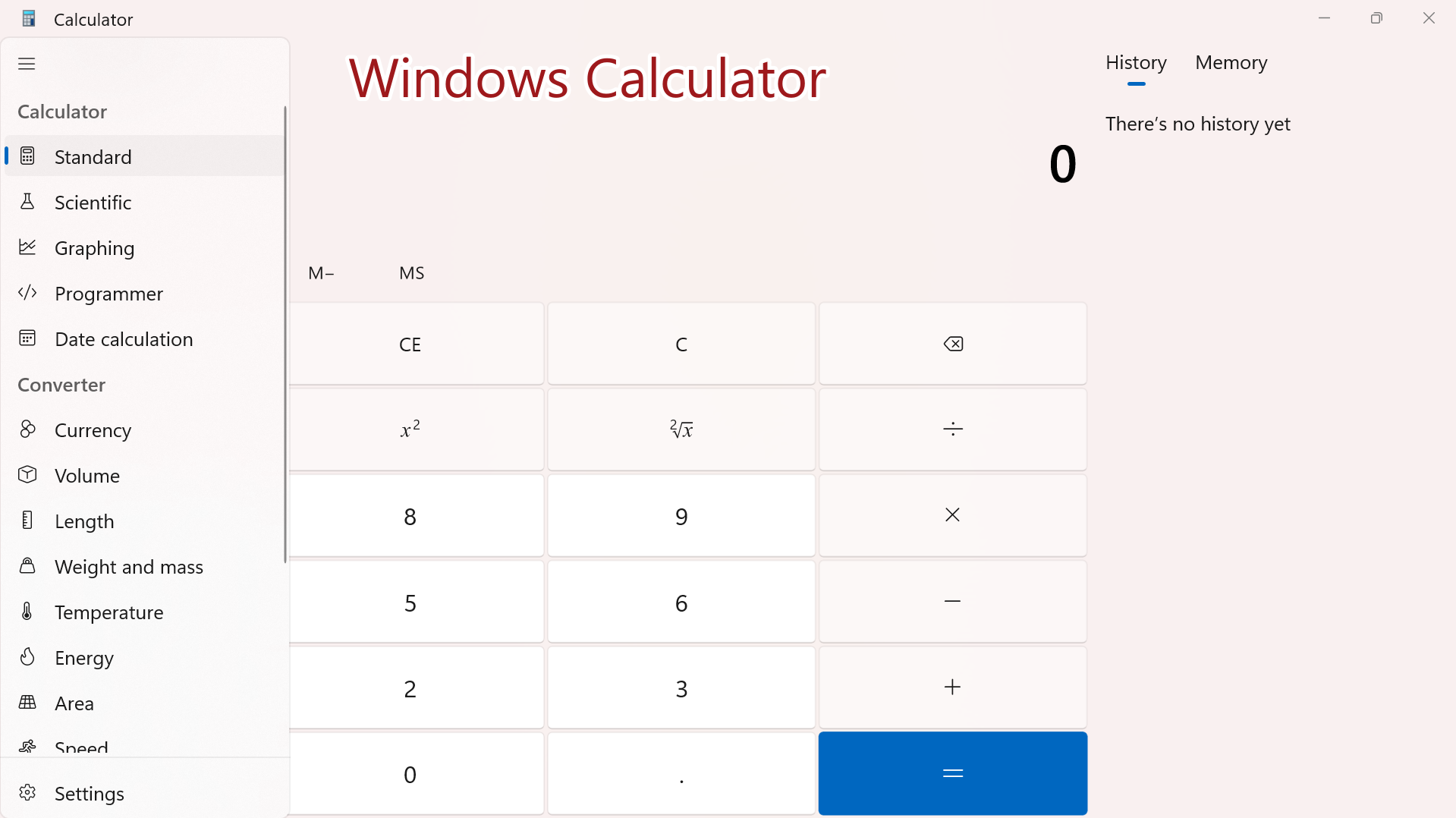### Testcase categories

https://www.testingdocs.com/how-to-write-test-cases/

## Functional Test Cases

A calculator is mostly used to perform mathematical calculations such as addition, subtraction, multiplication, division, etc.

Application Launch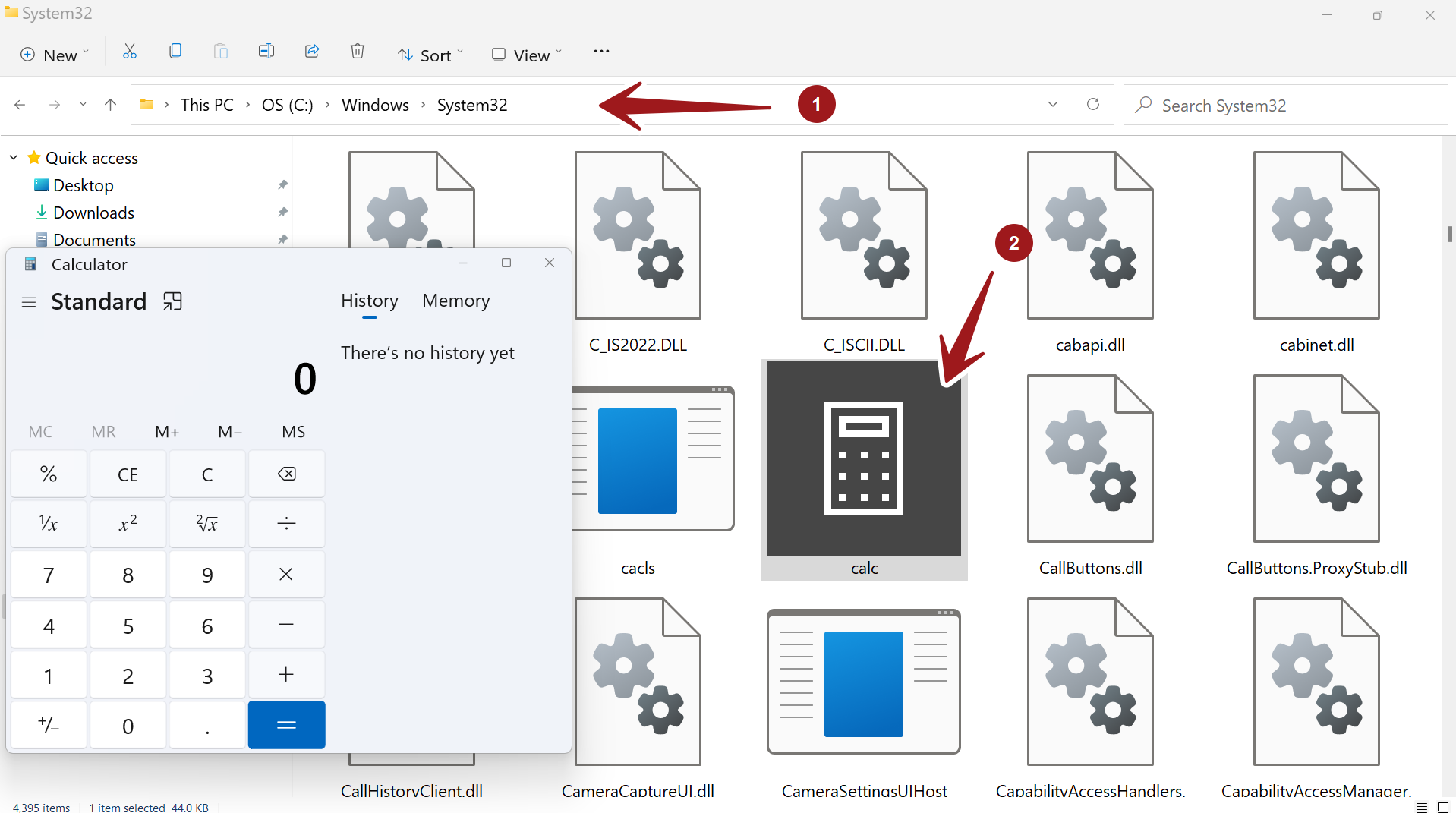Test Case Scenario Detailed Steps Test Environment & Data Expected Result Actual Result Verify Calculator Application Launch To verify that the Calculator application is launched successfully for use. Click on the Start menu icon and Choose Calculator. OR Open Run command prompt Change directory to System32 Type calc.exe Hit Enter button. Windows 10 The calculator should be launched successfully.

If this test case fails, it’s a show stopper and high priority defect(P1). Many test cases depend on this test case.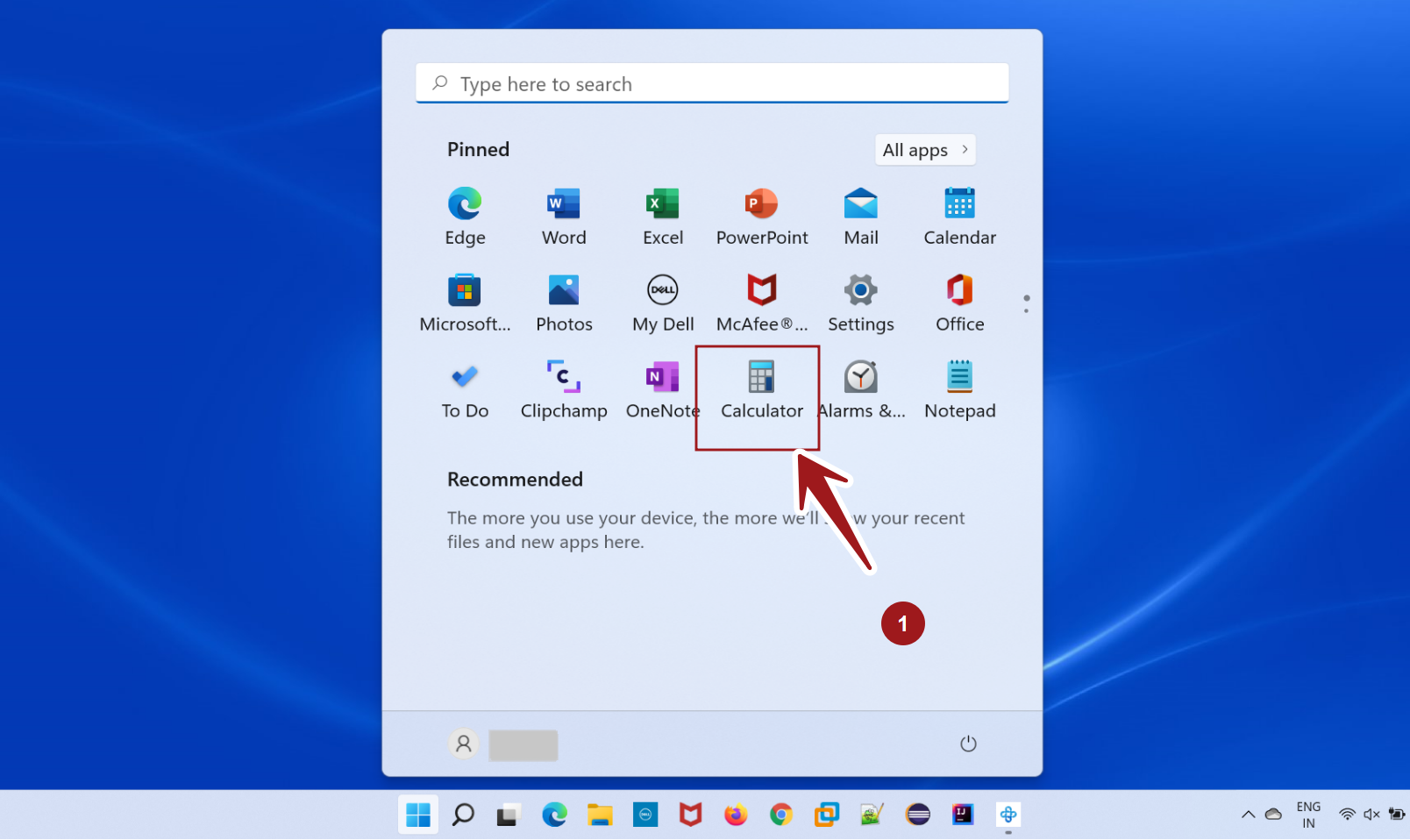### Basic Functionality Test Cases

These are the basic application test cases for the calculator. Button press, Clear screen, Memory Store, Memory Read,  Memory Remove Command History, etc.

 Test Case Scenario Detailed Steps Test Data Expected Result Actual Result Verify Button press. To verify that the Calculator application buttons are working as expected Launch Calculator application. Press any button. Watch the Calculator Screen. Press 9 button Should display 9 on the calculator screen.

## Core Mathematical Test Cases

Test the core mathematical functions like add, subtract, multiply, divide. etc.

 Test Case Scenario Detailed Steps Test Environment & Data Expected Result Actual Result Verify Add Function To verify the addition of numbers using the Calculator application. Launch Calculator Application. Enter the first number Click on the + button Enter the second number Click on the = button Windows 10 7 9 Verify that the sum of input numbers is displayed on the screen. 16 should be displayed on the screen.

Create test cases for other math functions like subtract, multiply, divide, square, percentage, and square root.

## BODMAS Rules

Verify mathematical expressions and adherence to BODMAS rules.

The BODMAS acronym stands for Brackets, Orders, Division, Multiplication, Addition, and Subtraction. These precedence rules should be applied while calculating the mathematical expressions.

Example:

( 2+ 7) * 3 + 9

=(9)*3+9 // Brackets first

= 27 + 9  // Multiplication takes precedence over addition

= 36

Note that: If addition takes precedence over multiplication which is against the BODMAS rule the result of the mathematical expression could be 108!

### Testing exceptions

One abnormal or exceptional test case is what happens when we divide any number by zero. Mathematically, the result is infinity. Since we don’t know the value the application should display an error message to the user.

 Test Case Scenario Detailed Steps Test Data Expected Result Actual Result Verify Divide by Zero To verify that Divide by zero operation. Launch Calculator application. Press 1 divide by 0. Watch the Calculator Screen. 1/0 Cannot divide by zero message.

## Profiling Calculator

Verify that all modules are loaded without any initialization errors.

Profile the Calculator application for DLL errors, loading and executing of modules, the occurrence of first chance exceptions, etc. with a profiler.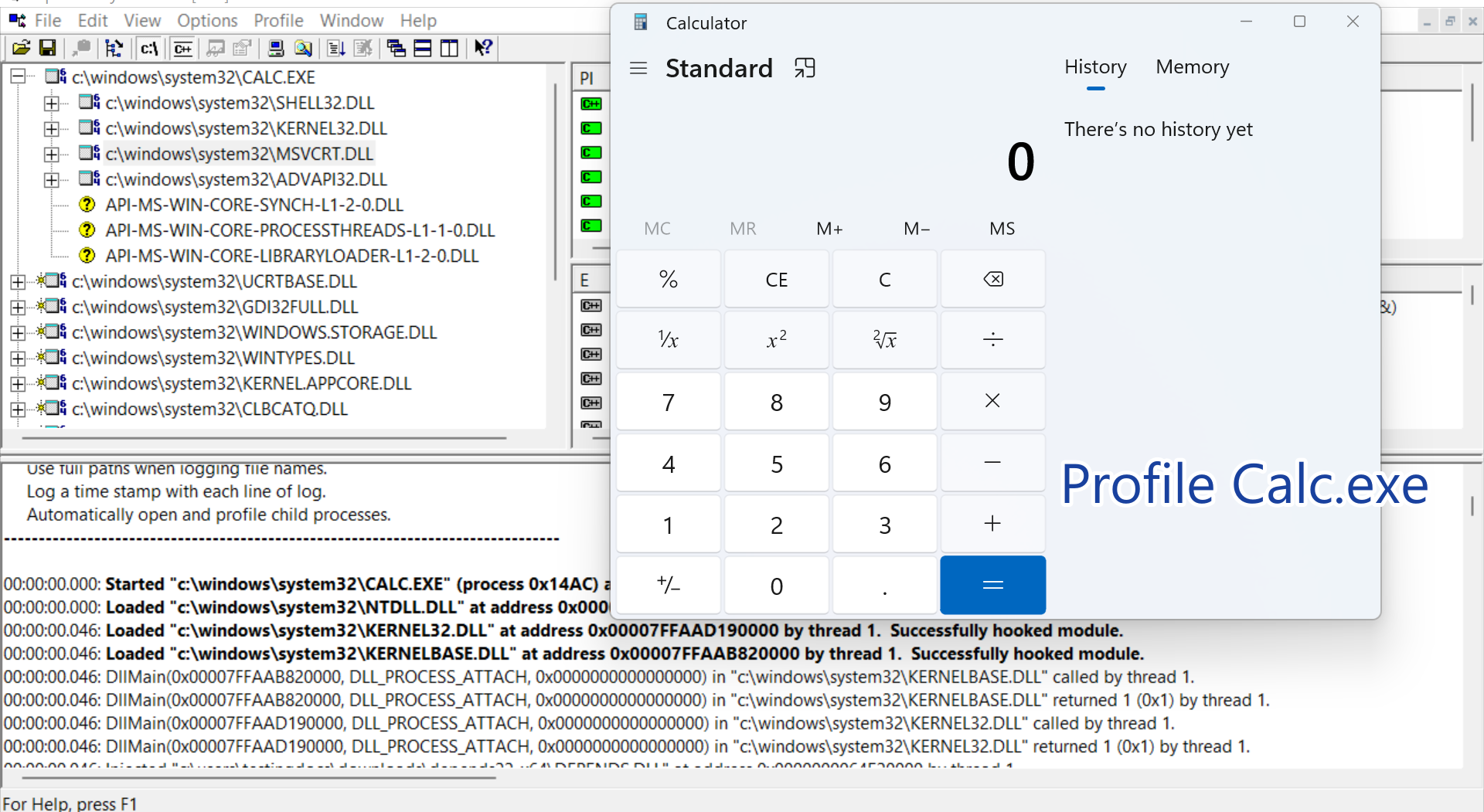### Uninstall Test Case

Verify that the Uninstall removes the Calculator App.

System -> Apps -> Apps & features -> Calculator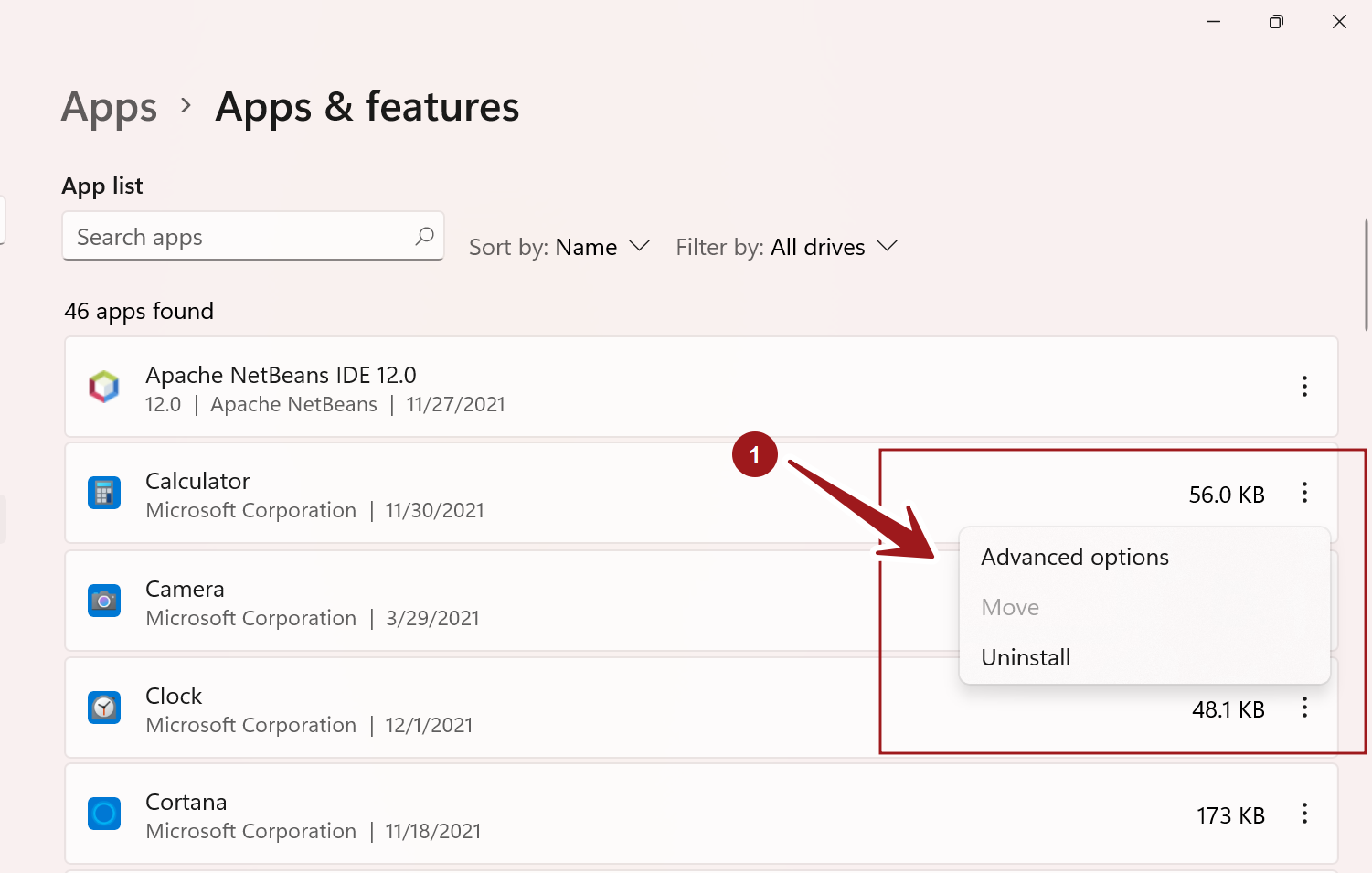## GUI and Look & Feel

These test cases are the usability and GUI test cases for the calculator application. Windows, Button colors combinations, easy of use like Start Menu launch, desktop shortcut, usability, and accessibility of the application.

For example, the button is accessible because the background color and the digit color are different and clearly visible. If the developer chooses the same color for both the button background and the digit color the button would not be accessible.

Verify the Application menu is working as expected.

For example, If the user selects the Scientific menu option, the Application should switch to Scientific mode.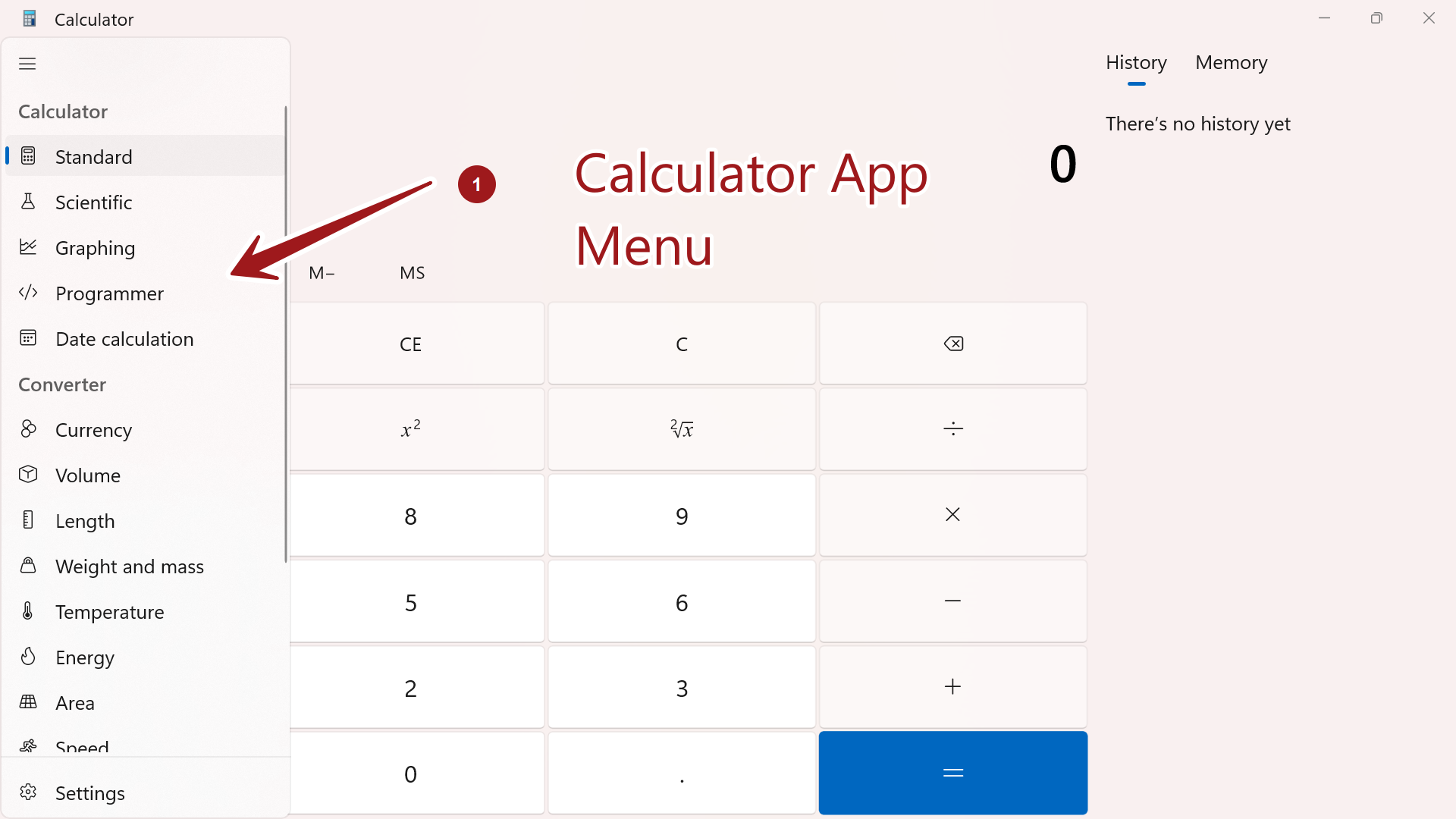## Usability

Application settings, Themes, Help, and Documentation for the end-user, Pin to Start Menu, Pin to Taskbar, etc.

Calculator UI Light Theme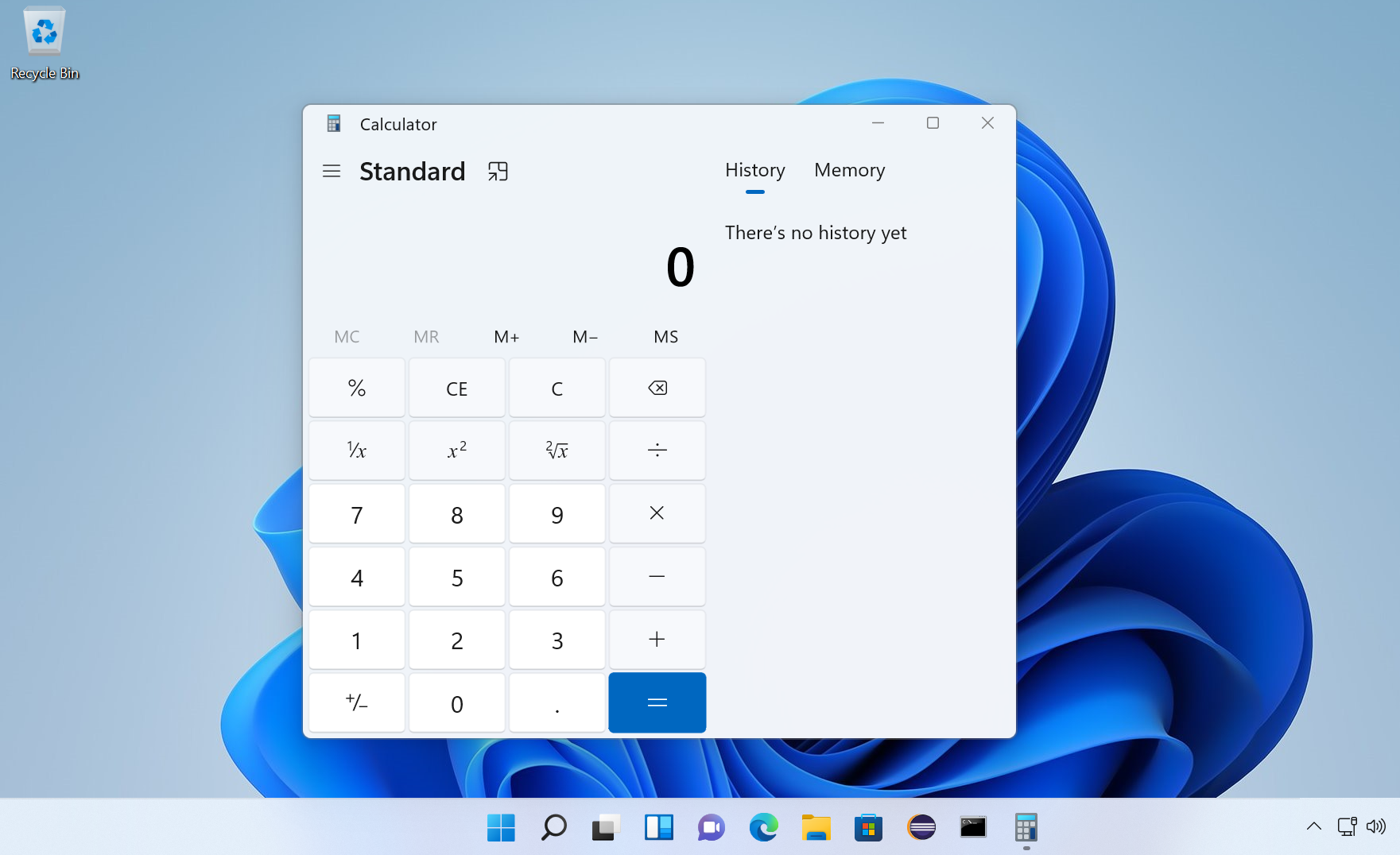Calculator UI Dark Theme

This theme emits less light and consumes less battery power on the PC.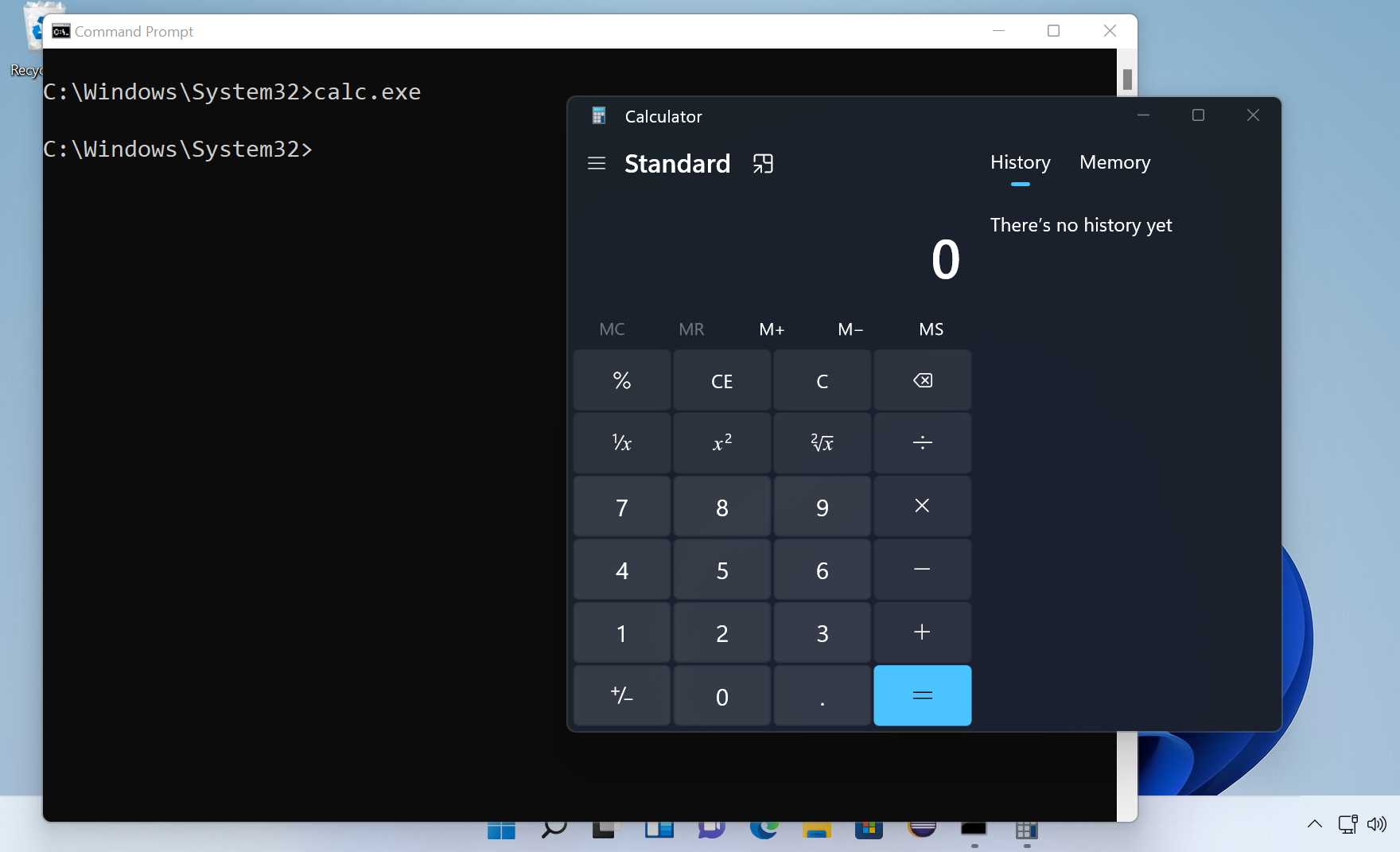Benchmark test:

Obtain two identical Windows machines, OEM reset both the machines. Charge the machines to 100% battery.  On one machine leave the Calculator with a Light theme and on another leave the Calculator running with a Dark theme.

Record the system uptimes.

### Help, Pin to Start Menu

Verify the Help documentation of the Calculator application.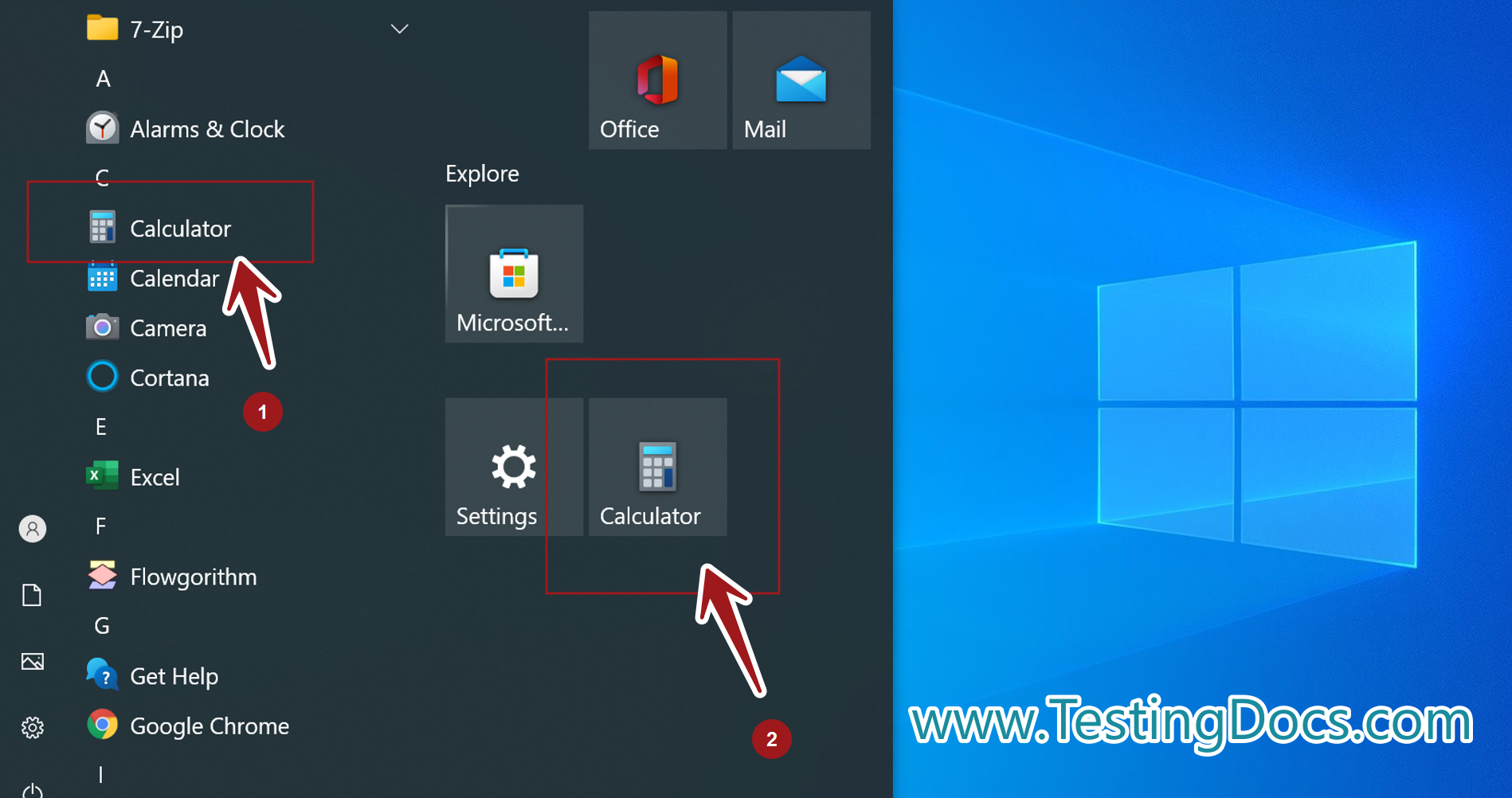## i18n and l10n Test cases

i18n stands for internationalization, and l10n stands for localization. These are the short forms of the words replacing the number of letters in between them.

i18n is customizing the application to many international languages. l10n is localizing the application to a particular locale.

Verify that the Calculator UI strings are localized in non-English locales. Install a non-English Windows OS, for example, Spanish (es) locale. Launch the Windows Calculator in a Spanish locale.

Verify that the UI is localized.

Verify that the strings are correctly localized(semantics) with language experts.

### Machine architectures

Verify the Calculator application on different machine processor architectures like 32-bit, 64-bit.

## Non-Functional Test Cases

Non-Functional test cases related to the calculator application. Some of the examples are performance, security, stress test cases, memory leak tests, etc.

Some examples for the test cases are:

What happens if the user leaves the application running for a long duration – does the application crash, leak memory, consume hardware resources, etc.

What happens when the user launches more than one instance of the application.

What happens when the user stores more calculations in the application. What is the limit?

The hardware footprint of the Calculator application in Task Manager application.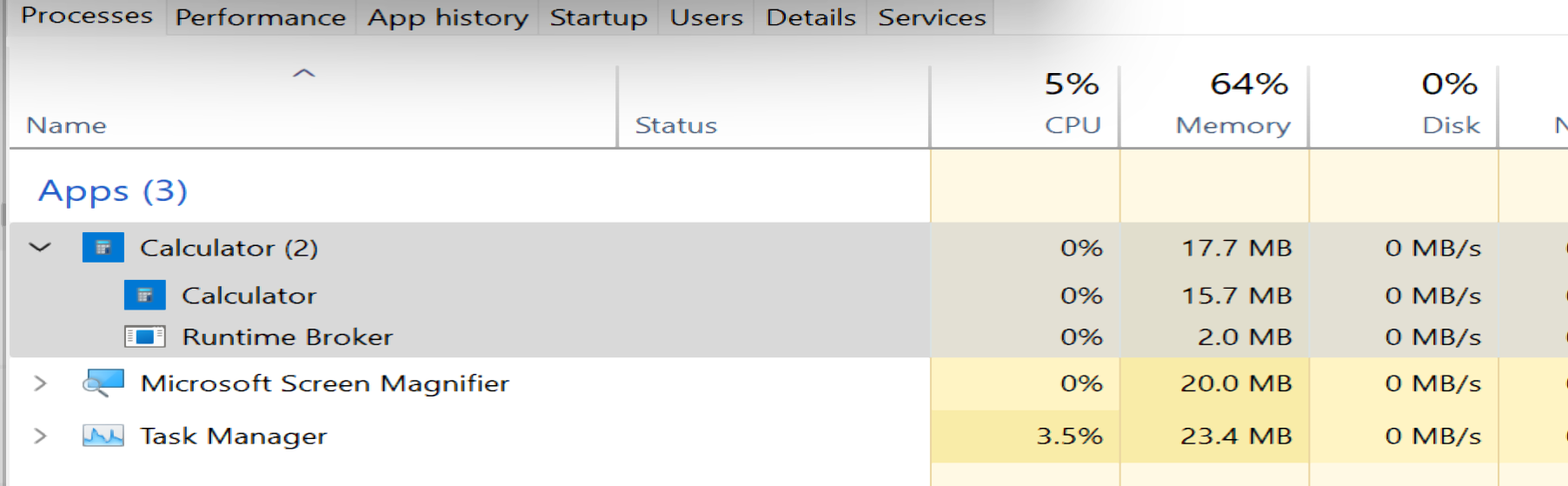## Endurance and Soak Test cases

These test cases stress the calculator application from normal working conditions. Example test cases

Soak Test Case

• Launch the Calculator Application.
• Leave the application running for a long period.
• Resume and perform some calculations.
• Verify that the application doesn’t crash.

Stress Test Case

• Launch multiple instances of the Calculator application. ( perhaps using an automation script)
• Randomly choose an instance and perform some calculations.
• Verify that the Calculator application is working.

## Compatibility Testing

Test the Calculator application on different screen resolutions and sizes.

Downgrade the operating system to Windows 7, 8  and test the backward compatibility.

Calculator on Windows 7 Operating System:Upgrade the operating system to Windows 11 Operating system and test the forward compatibility.### Automation

We can use tools like AutoIT to automate the Windows calculator.

Automated Unit Testing of Calculator Java class with JUnit testing framework:

https://www.testingdocs.com/junit-calculator-test-case-example/